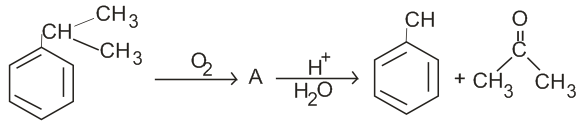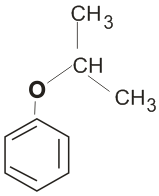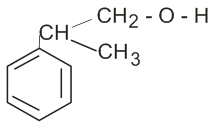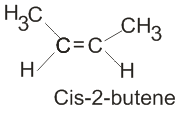## Filters

Sort by :
Q

Two similar thin equiconvex lenses , of focal length f each , are kept  coaxially in contact  with each other such that the focal length of the combination is  . When the space between the two lenses is filled  with glycerin  then the equivalent  focal length is  . The ratio

• Option 1)

2 : 1

• Option 2)

1 : 2

• Option 3)

2 : 3

• Option 4)

3 : 4

Option 1)2 : 1 Option 2)1 : 2 Option 3)2 : 3 Option 4)3 : 4

The non-essential amino acid among the following is:

• Option 1)

valine

• Option 2)

leucine

• Option 3)

alanine

• Option 4)

lysine

2

For an ideal solution, the correct option is:

• Option 1)

at constant T and P

• Option 2)

at constant T and P

• Option 3)

at constant T and P

• Option 4)

at constant T and P

For an ideal solution, the Gibbs free energy is always negative, thus their mixing is always spontaneous. The volume change of the mixing of ideal solutions is zero. An ideal solution is one in which the enthalpy of the solution is zero  at constant T and P Option 3 is correct.Option 1) at constant T and POption 2) at constant T and POption 3)  at constant T and POption 4) at constant T and P

For the cell reaction

at 298K. The standard Gibbs energy  of the cell reaction is:

[Given that Faraday constant F = 96500 C mol-1]

• Option 1)

-46.32 kJ mol-1

• Option 2)

-23.16 kJ mol-1

• Option 3)

46.32 kJ mol-1

• Option 4)

23.16 kJ mol-1

We know that       Option 1 is correctOption 1)-46.32 kJ mol-1Option 2)-23.16 kJ mol-1Option 3)46.32 kJ mol-1Option 4)23.16 kJ mol-1

Enzymes that utilize ATP in phosphate transfer require an alkaline earth metal (M) as the cofactor M is:

• Option 1)

Be

• Option 2)

Mg

• Option 3)

Ca

• Option 4)

Sr

Option 2 Mg is the alkaline earth metal as the co-factor. (FACT)Option 1)BeOption 2)MgOption 3)CaOption 4)Sr

A compound is formed by cation C and anion A. The Anions form hexagonal close packed lattice and the cations occupy 75% of the octahedral void. The formula of the compound is

• Option 1)

• Option 2)

• Option 3)

• Option 4)

Option 3 Anions: No of ions per unit in HCP = 6 Cations: No of octahedral voids in HCP = 6                         So, 75% of 6 = 4.5     Option 1)Option 2)Option 3)Option 4)

A gas at 350K and 15 bar has molar volume 20 percent smaller than that for an ideal gas under the same conditions. The  correct  option about the gas and its compressibility factor (Z) is:

• Option 1)

Z>1 and attractive forces are dominant

• Option 2)

Z>1 and repulsive forces are dominant

• Option 3)

Z<1 and attractive forces are dominant

• Option 4)

Z<1 and repulsive forces are dominant

Option 3          Molar volume of gas is 20% smaller than that of the ideal gas. Hence Z < 1 and attractive forces are dominant. When Z < 1: Gas shows negative deviation and is more compressible than expected from ideal behaviour.  Z < 1 corresponds to attractive forces between molecules.Option 1)Z>1 and attractive forces are dominantOption 2)Z>1 and repulsive forces are dominantOption 3)Z<1...

• Option 1)

nylon-6,6

• Option 2)

nylon 2 - nylon 6

• Option 3)

nylon-6

• Option 4)

Buna-S

Option 2  Biodegradable polymer is Nylon-2-Nylon-6 monomers: Glycine, aminocaproic acid Option 1)nylon-6,6Option 2)nylon 2 - nylon 6Option 3)nylon-6Option 4)Buna-S

For the chemical reaction

the correct option is:

• Option 1)

• Option 2)

• Option 3)

• Option 4)

For a chemical reaction: The rate is always given as:  for the reaction: , Thus,  Option 3 is correct.Option 1)Option 2)Option 3)Option 4)

The total energy of an electron in an atom in an orbit is -3.4 eV . Its kinetic and potential energies are respectively

• Option 1)

-3.4 e V, -3.4 eV

• Option 2)

-3.4 e V , - 6.8 e V

• Option 3)

3.4 e V , - 6.8 e V

• Option 4)

3.4 e V , 3.4 e V

Option 1) -3.4 e V, -3.4 eV  Option 2) -3.4 e V , - 6.8 e V  Option 3) 3.4 e V , - 6.8 e V  Option 4) 3.4 e V , 3.4 e V

Under isothermal condition, a gas at 300K expands from 0.1L to 0.25L against a constant external pressure of 2 bar. The work done by the gas is:

[Given that 1L bar = 100 J]

• Option 1)

-30 J

• Option 2)

5 kJ

• Option 3)

25 J

• Option 4)

30 J

We know that the work done is given as: Now, Option 1 is correct.Option 1)-30 JOption 2)5 kJOption 3)25 JOption 4)30 J

A soap bubble , having radius of 1 mm is blown from, a detergent solution having a surface tension , of . The pressure inside the bubble equals at a point  below  the free surface of water in a container of water =  , the value of  is

• Option 1)

100 cm

• Option 2)

10 cm

• Option 3)

1 cm

• Option 4)

0.5 cm

GIVEN : surface tension = force /length = 0.025 N/m

p(final) - p(initial) = 4t/r

where z is a pressure inside the bubble

p(z=final) - p(initial) =pgh =pgz = 4t/r

pgz = 4t/r

z = 4t/pgr

z = 4(0.025)/(0.001)(1000)(10)

z =0.100/10

z = 0.100(0.1)

z = 0.01

z= 1(0.01)

z=1cm

option 3) 1cm

View More

Which of the following reactions are disproportionation reaction?

(a)

(b)

(c)

(d)

Select the correct option from the following:

• Option 1)

(a) and (b) only

• Option 2)

(a), (b) and (c)

• Option 3)

(a), (c) and (d)

• Option 4)

(a) and (d) only

Option 1 Disproportionation is a type of redox reaction in which a species is simultaneously reduced and oxidised forming two different products.  (a)  (b) Option 1)(a) and (b) onlyOption 2)(a), (b) and (c)Option 3)(a), (c) and (d)Option 4)(a) and (d) only

If the rate constant for a first order reaction is k, the time (t) required for the completion of 99% of the reaction is given by:

• Option 1)

t = 0.693/k

• Option 2)

t = 6.909/k

• Option 3)

t = 4.606/k

• Option 4)

t = 2.303/k

Option 3 For first order reaction,               Option 1) t = 0.693/k Option 2) t = 6.909/k Option 3) t = 4.606/k Option 4) t = 2.303/k

The structure of intermediate A, in the following reaction, is:• Option 1)• Option 2)• Option 3)• Option 4)Option 2   Cumene is oxidized in air, which removes the tertiary benzylic hydrogen from cumene and hence forms cumene radical. The cumene radical then bonds with an oxygen molecule to give cumene peroxide radical, which in turn forms cumene hydroperoxide by abstracting benzylic hydrogen from the cumene molecule.  Option 1)Option 2)Option 3)Option 4)

Among the following, the reaction that proceeds through an electrophilic substitution is:

• Option 1)• Option 2)• Option 3)• Option 4)The electrophilic substitution reaction is: Option 2 is correct  Option 1)Option 2)Option 3)Option 4)

The most suitable reagent for the following conversion is:• Option 1)

Na/liquid NH3

• Option 2)

H2, Pd/C, Quinoline

• Option 3)

Zn/ HCl

• Option 4)

Hg2+/H+, H2O

Option 1)Na/liquid NH3Option 2)H2, Pd/C, QuinolineOption 3)Zn/ HClOption 4)Hg2+/H+, H2O

The manganate and permanganate ions are tetrahedral, due to:

• Option 1)

The -bond involves overlap of p-orbitals of oxygen with d-orbitals of manganese.

• Option 2)

There is no -bonding.

• Option 3)

The -bonding involves the overlap of p-orbitals of oxygen with p-orbitals of manganese

• Option 4)

The bonding involves overlap of d-orbitals of oxygen with d-orbitals of manganese.

Option 1 Manganate ion:-   [Green, paramagnetic] Permanganate ion:-  [purple, diamagnetic]   These ions are tetrahedral in shape. The -bonding takes place by overlap of p-orbitals of oxygen with d-orbitals of manganese.Option 1)The -bond involves overlap of p-orbitals of oxygen with d-orbitals of manganese.Option 2)There is no -bonding.Option 3)The -bonding involves the overlap of p-orbitals of...

The unit of thermal conductivity is :

• Option 1)

• Option 2)

• Option 3)

• Option 4)

Option 1)Option 2)Option 3)Option 4)

Which of the following diatomic molecular species has only -bonds according to Molecular Orbital Theory?

• Option 1)

O2

• Option 2)

N2

• Option 3)

C2

• Option 4)

Be2

Molecular orbital diagram for C2:  both are  bondsOption 1)O2Option 2)N2Option 3)C2Option 4)Be2
Exams
Articles
Questions